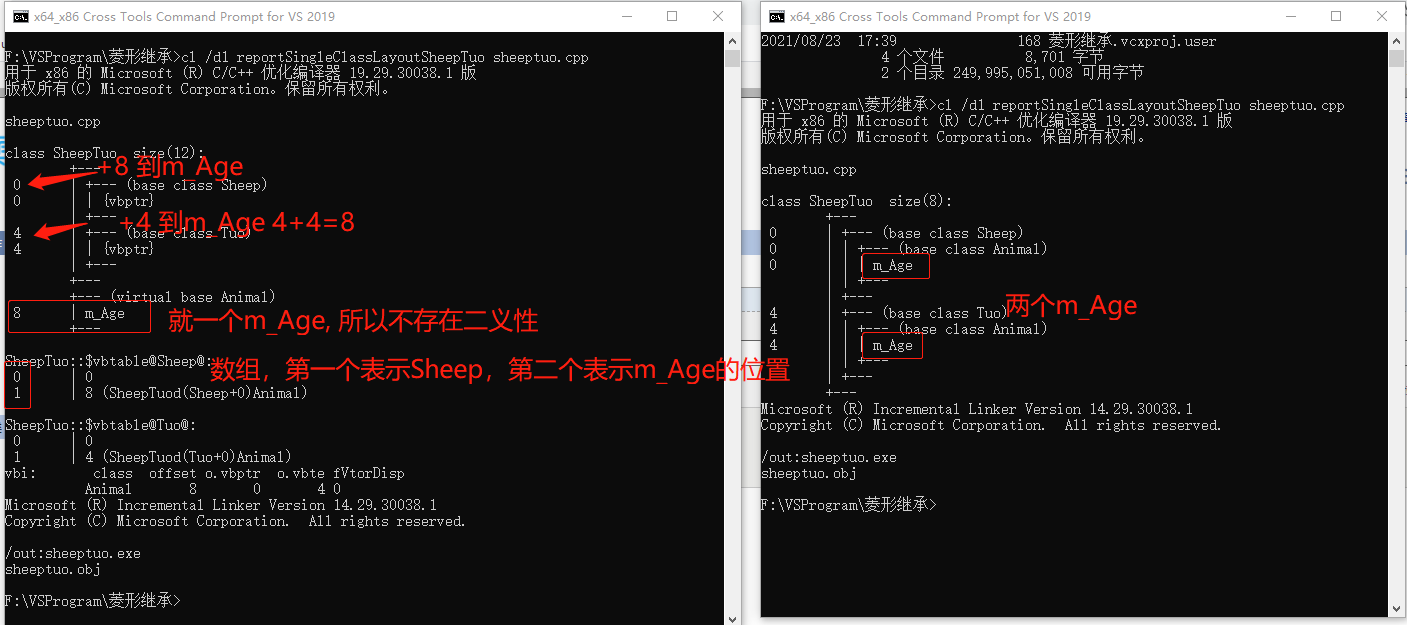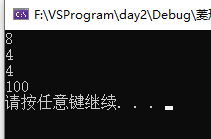# 导航

1。对照表来看#define _CRT_SECURE_NO_WARNINGS
#include <iostream>
using namespace std;

class Animal
{
public:

int m_Age;
};
//虚基类 Sheep
class Sheep:virtual public Animal
{
};
//虚基类 Tuo
class Tuo: virtual public Animal
{};
class SheepTuo :public Sheep, public Tuo
{
};
void test01()
{
SheepTuo st;
st.Sheep::m_Age = 10;
st.Tuo::m_Age = 20;

cout << st.Sheep::m_Age << endl;    //20 因为指向的时同一个地址
cout << st.Tuo::m_Age << endl;  //20
cout << st.m_Age << endl;   //20 可以直接访问m_Age数据 原因时因为没有二义性了
}
//通过地址 找到偏移量
//内部工作原理
void test02()
{
SheepTuo st;
st.m_Age = 100;
//找Sheep的偏移量操作
// &st 对照类的模型表来看 先取到地址
//(int *)&st //转成int* 是为了改变步长
// *(int *)&st  //取* 找到虚基类表
// (int *) * (int *)&st  //去到虚基类表的步长
// (int *) * (int *)&st + 1  //取到虚基类表的步长 + 1 获取到Sheep数组的第1位
// （int *）((int *) * (int *)&st + 1)  //取到步长后再加int *  此时地址指向偏移量所在的位置
// *（int *）((int *) * (int *)&st + 1)  //取到数据所在的位置 前面加* 获取到值 如图所以 Sheep的数组为 0：0， 1：8
cout << *(int*)((int*)*(int*)&st + 1) << endl;  //获取到Sheep的偏移量为 8

//找Tuo的偏移量
//(int *)&st + 1;   //先取到地址 改变步长后 找Tuo虚基类表的位置为 改变步长后+1 找到Tuo的虚基类表的地址
// *((int *)&st + 1)  //根据Tuo的虚基类表的地址取* 找到Tuo的虚基类表
// (int*)*((int *)&st + 1) //根据Tuo虚继类表取步长 获取数组的地址
// *((int*)*((int *)&st + 1)+1)  //根据数组的地址 取第1位的地址 取* 获得偏移量
cout << *((int*)*((int*)&st + 1) + 1) << endl;  //获取Tuo的便宜量为 4
cout << *((int*)((int*)*((int*)&st + 1) + 1)) << endl; //教程为这个 答案也是4

//输出m_Age
//  (char *)&st是 0的位置 + 8  然后强转成Animal类
cout << ((Animal*)((char*)&st + *(int*)((int*)*(int*)&st + 1)))->m_Age << endl;

}
int main()
{
test02();
//test01();
system("Pause");
return 0;
}posted on 2021-08-23 20:40  BZ易风  阅读(56)  评论(0编辑  收藏  举报